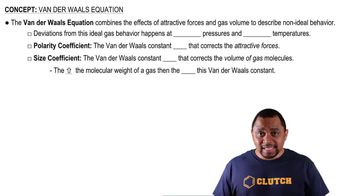Start typing, then use the up and down arrows to select an option from the list.
7:54 minutes
Problem 94b
Textbook Question

# Use the van der Waals equation and the ideal gas equation to calculate the pressure exerted by 1.000 mol of Cl2 in a volume of 5.000 L at a temperature of 273.0 K. Explain why the two values are different.Verified Solution
This video solution was recommended by our tutors as helpful for the problem above.
474views

### Watch next

Master Van der Waals Equation with a bite sized video explanation from Jules Bruno

Start learning01:4003:1903:55